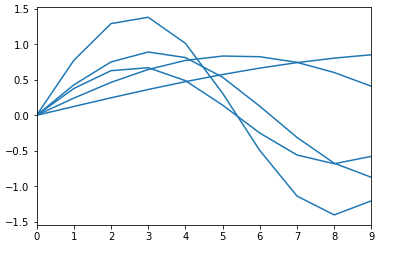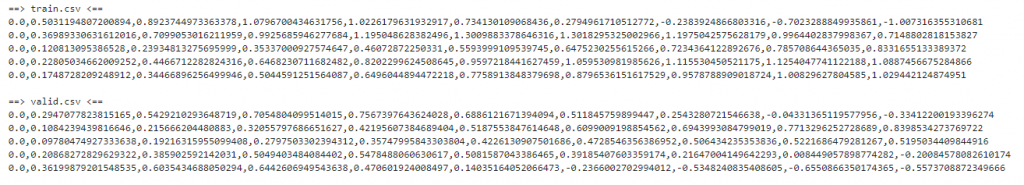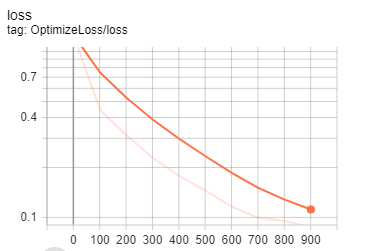DAY 30
0

# Art and Science of Machine Learning

## Custom Estimator

### Using Custom Estimators

1. 首先第一步當然就是安裝相關套件，並且設定相關位置與資訊，進行環境的建置。
``````import os
PROJECT = 'xxx' #改成自己的資訊
BUCKET = 'xxx'
REGION = 'xxx'
``````
1. 接著利用隨機的方式產生數列，可以看到這邊y的範圍從正負1.5，我們的目標想要預測的是在下一個時間點(x=10)時結果為何，並且將這些隨機產生的資料寫入csv檔中做訓練與驗證集2. 定義一個function去讀取csv，並且將數據的前面去除最後一位做為輸入資料、最後一位設定為labels，透過批次化的處理讀取檔案

``````inputs = all_data[:len(all_data) - N_OUTPUTS]
labels = all_data[len(all_data) - N_OUTPUTS:]
``````
3. 建構RNN模型

• 定義RNN基礎架構
``````lstm_cell = rnn.BasicLSTMCell("層數", forget_bias = "bias")
outputs, _ = rnn.static_rnn(lstm_cell, "輸入資料", dtype = tf.float32)
``````
• 定義loss function、優化器、評估方式
``````loss = tf.losses.mean_squared_error(labels, "預測結果")
train_op = tf.contrib.layers.optimize_loss
(loss = loss,learning_rate = 0.01,optimizer = "SGD")
eval_metric_ops = {"rmse": tf.metrics.root_mean_squared_error(labels, "預測結果")}
``````
• 創造預測字典與建立輸出位置
``````predictions_dict = {"predicted": 預測結果}
export_outputs = {"predict_export_outputs": tf.estimator.export.PredictOutput(outputs = predictions)}
``````
• 最後回歸所有資訊
``````return tf.estimator.EstimatorSpec(
predictions = predictions_dict,loss = loss, train_op = train_op, eval_metric_ops = eval_metric_ops,export_outputs = export_outputs)
``````
4. 使用Estimator API來載入我們剛剛建構的模型

``````estimator = tf.estimator.Estimator(model_fn = "模型副程式",  model_dir = "輸出資料夾")
``````
5. 最後可以決定要再本地端進行訓練或是使用Cloud ML Engine，唯一不同的地方只有需要更改路徑以及相關bucket的設定，並且可以透過TensorBoard來觀察訓練成效6. 另外這些架構也都可以利用keras來做實作，keras有點像堆疊的概念，將其架構排列組合形成我們所要的功能

``````model = keras.models.Sequential()
model.compile(loss = 'mean_squared_error', optimizer = 'adam',metrics = ['mae', 'mape'])
# LOSS function 、優化器、評估模式
``````